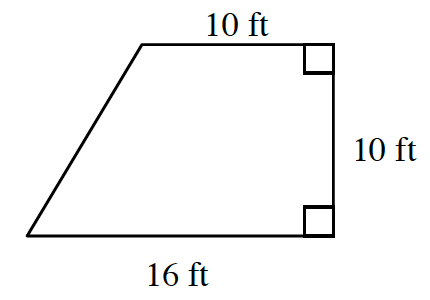Home > CC2MN > Chapter cc24 > Lesson cc24.3.1 > Problem4-78

4-78.Dante is ordering wood flooring for his bedroom, which is shaped like a trapezoid (shown below). If the flooring materials cost $\5$ per square foot, how much should he expect to pay for the materials? Homework Help ✎

First, you must find the area of the trapezoid, since this in the amount of flooring material Dante will need.

$\frac{1}{2} (10)(16 + 10) = 5(26) = 130$ square feet

Next, multiply the area by $\5$ to find the cost of the necessary flooring materials. $(130)(5)=\650$

$\650$